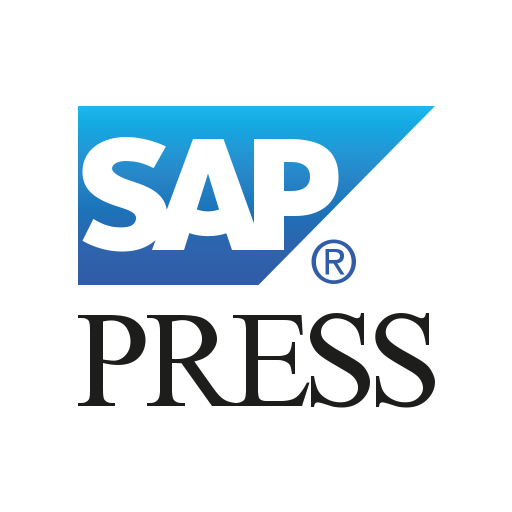R functions can be called from SAP HANA using a stored procedure with the RLANG language. Specific R codes to be executed are written within this procedure.

You can call this procedure from the SQL Editor or call it from a wrapper stored procedure written in SQLScript. Additionally, R functions can be called from flowgraphs using AFM tools. This blog post discusses the different available options with sample data and code for better understanding. All the codes used in this post are available in text format at this website: www.sap-press.com/5014. (Go to Product Supplements, download the .zip file and open the Code > Chapter 6 file.)

We’ll go ahead and use table LM_INPUT_DATA as the historical data for modeling and table LM_NEW_DATA as the new data for prediction.

Like SAP HANA PAL procedures, you’ll need to provide some input tables for this procedure, and the procedure will return the result through some output tables. These input and output tables are passed as IN and OUT parameters of the procedure. However, unlike SAP HANA PAL procedures, no fixed number or structure for input and output tables is expected. The structure will solely depend on how you want to pass the data to the procedure and how you want to retrieve the results.

6

The data exchange between SAP HANA and R takes place only through SAP HANA tables and R data frames. Input tables passed from SAP HANA are read as data frames in R. The output in R should be prepared as data frames, which will be read as tables by SAP HANA.

We’ll use the following tables as input and output tables in SAP HANA:

• Input table
• LM_INPUT_DATA: The data we’ll use for modeling purpose.
• Output tables
• LM_MODEL_COEFFICIENTS: To store the model coefficients of regression analysis.
• LM_MODEL_STATISTICS: To store the statistics related to model fitting.
• LM_FITTED: To store the fitted values of dependent variables (y).

Now, we’re all set to write our procedure in RLANG language. The following figure shows the procedure in SAP HANA that embeds the associated R codes.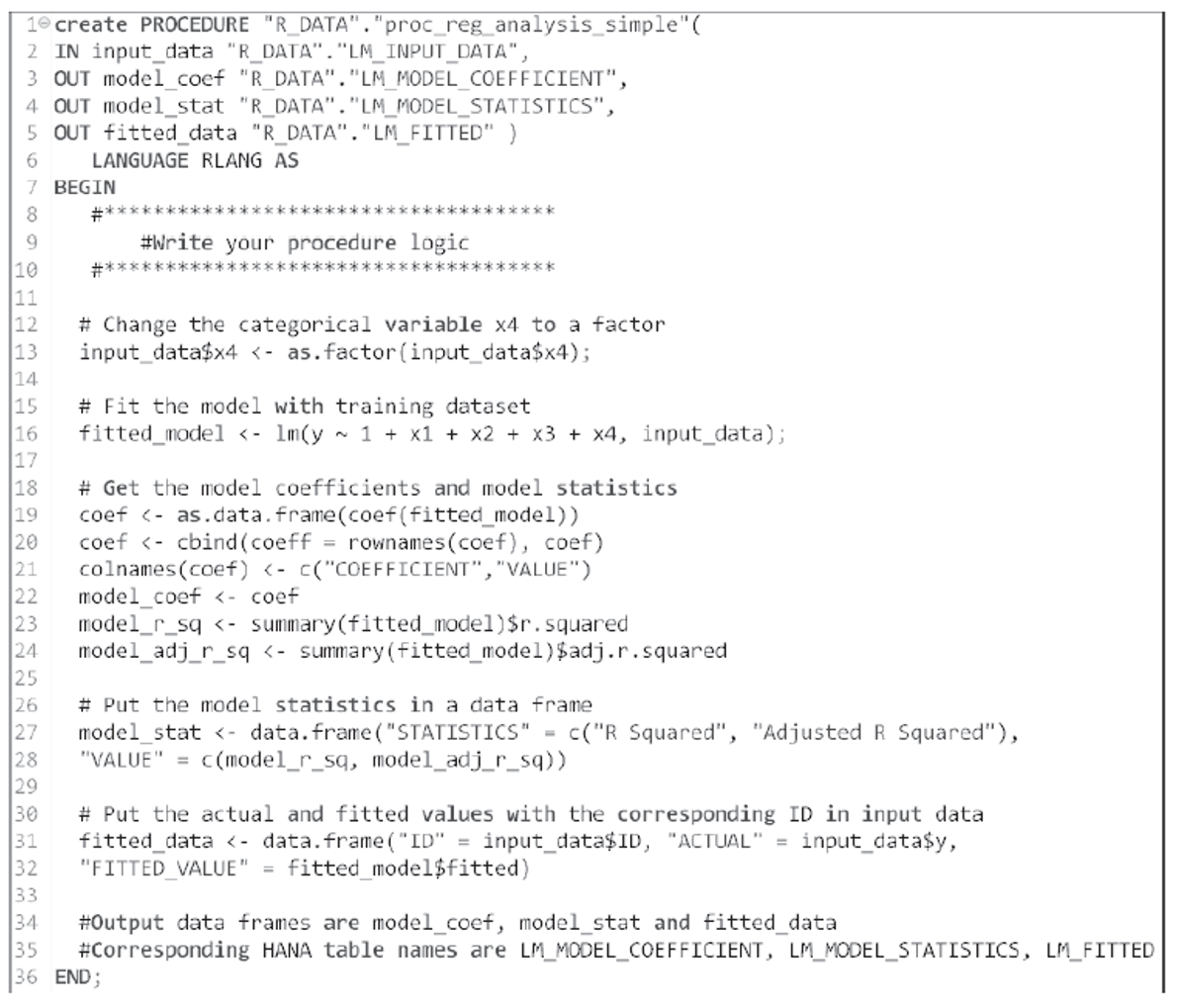Note that x4 is a categorical variable, so we cast it as a factor. Including this step is a good practice, since categorical variables with numeric values will be treated as continuous variables unless explicitly converted into factors.

We’ve fitted our model using the lm() function of R. This function fits a multiple linear regression of y on x1, x2, x3, and x4 with the intercept term. The executed model is assigned to an object called fitted_model, which is the reference of our model for future use.

The model coefficients and statistics are provided by the lm() function. We used R codes to retrieve and store specific values in data frames. At first, the R code looks complex, but with a little practice, writing this code will become pretty simple.

We’ll need to call this procedure to execute it to get the output. You can call this procedure either from the SQL Editor or from an SQLScript procedure.

## From the SQL Editor

You can run the code shown in the figure below via the SQL Editor to call the procedure.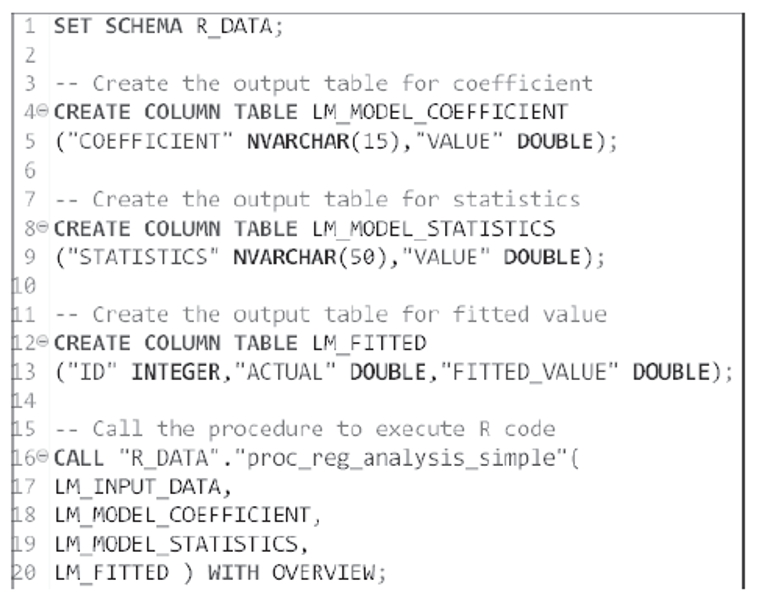The procedure proc_reg_analysis_simple runs the R scripts and stores the results in the output tables. After successful execution, the SQL Editor will display the table shown here, which includes the mapping between the parameter and output tables.

6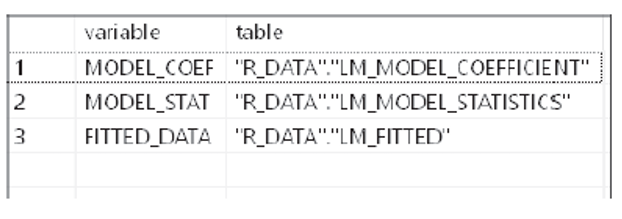The output of the multiple linear regression algorithm is stored in the output tables, as shown in the next three figures.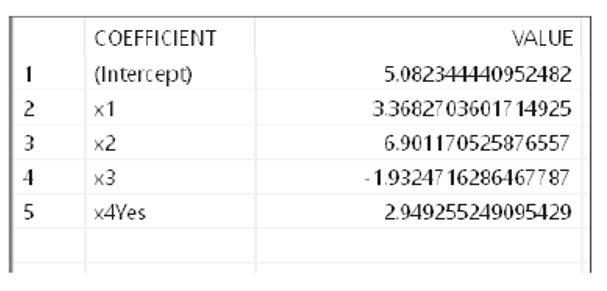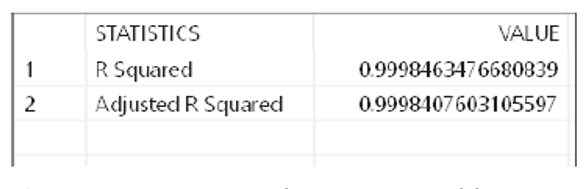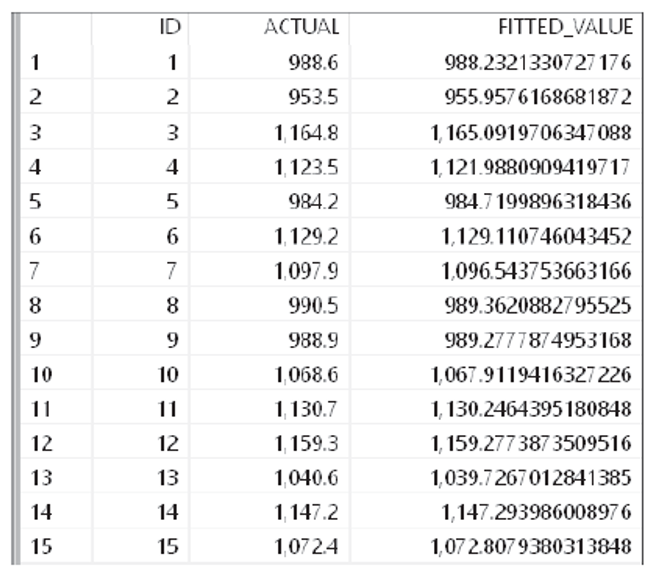• R encoded the x4 column with “Yes” as 1 and “No” as 0. Thus, the coefficient table shows coefficient associated with the “Yes” value only.
• The statistics table is showing the two statistics we specified in the R code. However, we can get many other statistics from R if required.
• As per our R code, the fitted table shows both actual and fitted values of y side by side.

## Calling from an SQLScript Procedure

Once our initial model exploration is complete, we can store the entire logic in an SQLScript procedure and call the RLANG procedure from this wrapper procedure. The code shown in this figure is an example of using this wrapper.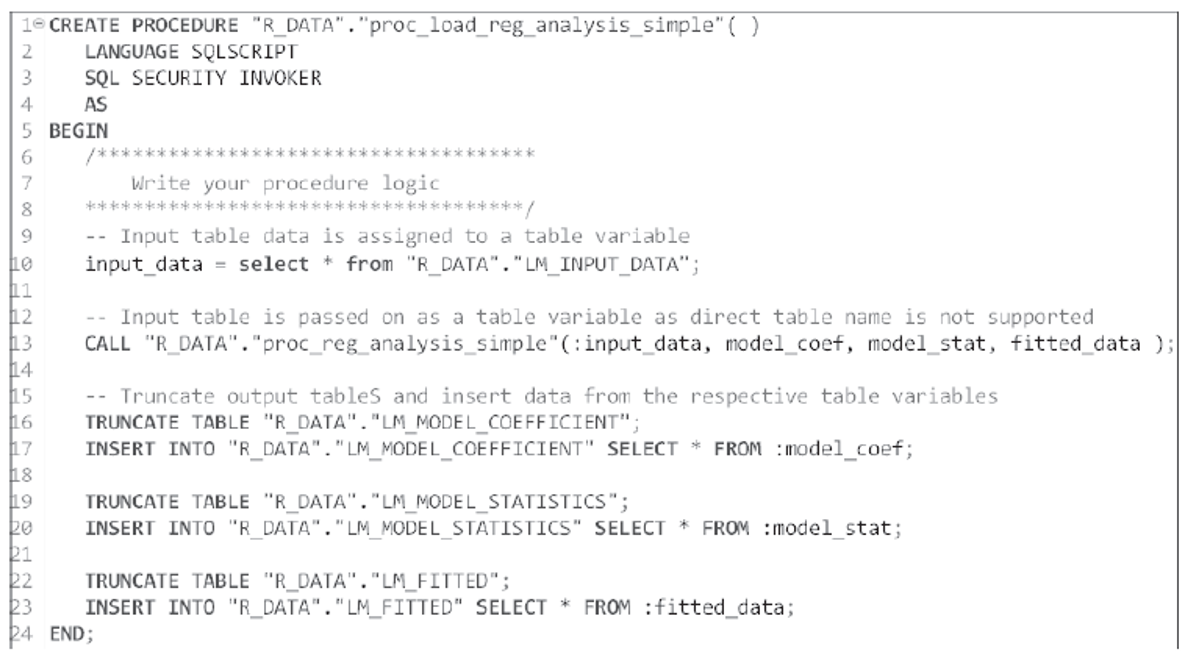• We passed the input data (LM_INPUT_DATA) through a table variable.
• The output generated by the RLANG procedure is returned as table variables. We’ll use these values to store the data in the relevant tables.
• Since the output tables are already defined, we haven’t defined them here. We truncated these tables to remove any existing records.

The SQLScript procedure can be executed by using the following code:

`Call “R_DATA”.”proc_load_reg_analysis_simple”();`

The RLANG procedure will be executed, and its output will be inserted into the output tables. Of course, the output tables should have the same data that we got previously through the SQL Editor.

You can use the R model (fitted_model) for prediction purposes also. This figure shows the associated RLANG procedure.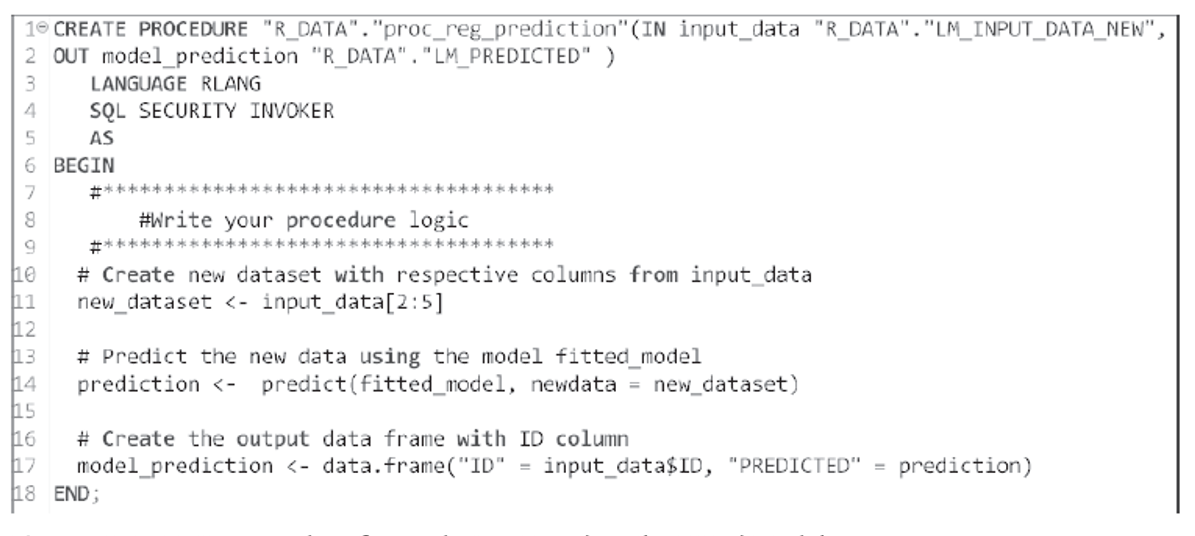Note the following:

• In this case, we passed the new data (table LM_INPUT_DATA_NEW) as the input data for prediction. We removed the ID column before prediction since we don’t need an ID value for our prediction.
• All the model information is already stored in the object fitted_model.
• The output is stored in a new table with two columns: ID (to store the IDs) of the records and PREDICTED (to store the predicted values of y).

The SQLScript wrapper procedure shown in this figure can be used to call this RLANG procedure.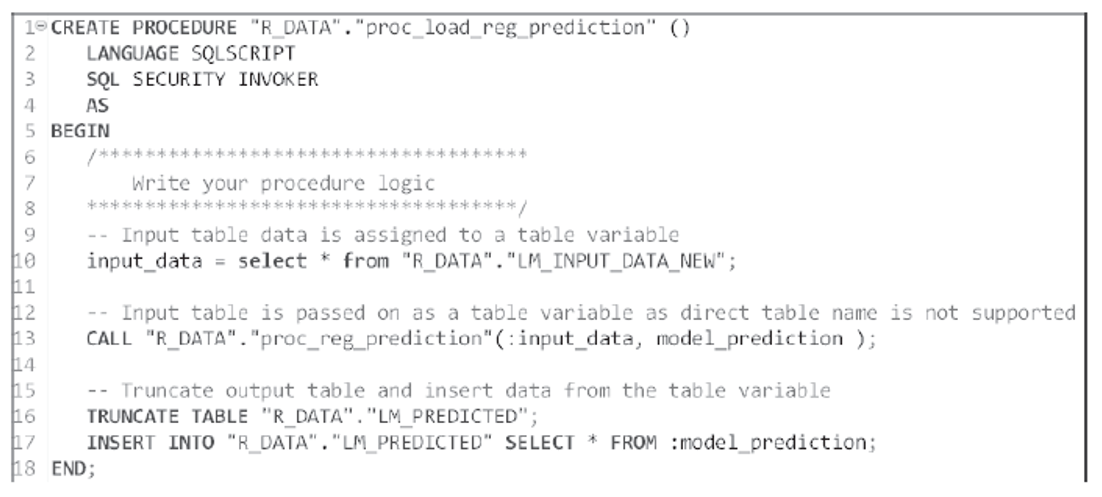The output table contains the ID and the predicted value of y. The following figure shows the contents of this table.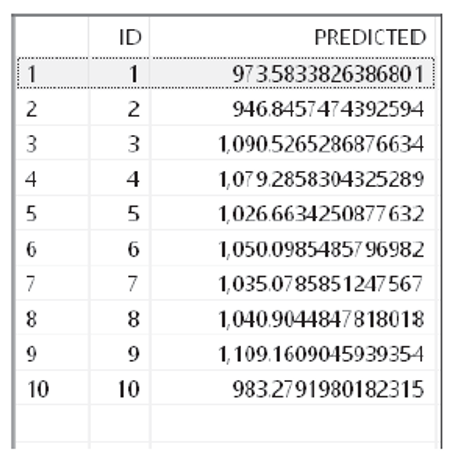## Saving and Retrieving R Models

We used the predefined model in R for prediction purposes. However, another user may run their own model in R and store the model information in the same fitted_model object. Then, all our predictions will be wrong. Thus, you can store the model information and retrieve it during prediction in one of two ways:

1. Save the model in R as a R file and load it when needed. The R code save(fitted_model, file = "my_lm_model.rda") saves the fitted_model in an .rda file in the working directory. During prediction, the fitted_model object can be retrieved from the file using R code load("my_lm_model.rda"), which will reload the fitted_model
2. You can serialize the fitted_model object in Raw data type and send this to SAP HANA. The object will be stored as the BLOB data type in SAP HANA. During prediction, you can send this BLOB data to back R and unserialize it in R to restore this version f the fitted_model Please refer to SAP HANA R Integration Guide from SAP for an example (http://s-prs.co/501403).

## From Flowgraphs

Like SAP HANA PAL functions, flowgraphs can also be used to call R scripts using the R Script node template. The R code can be written under the Script tab of the Properties view. SAP HANA also provides example codes in the R Examples section of the Node Palette.

## Conclusion

In this post, we showed you how to call R functions from SAP HANA in a number of different ways. We hope it helps you as you work on developing some intelligent technologies for your business!

Editor’s note: This post has been adapted from a section of the book Machine Learning with SAP: Models and Applications by Laboni Bhowmik, Avijit Dhar, and Ranajay Mukherjee.

## Recommendation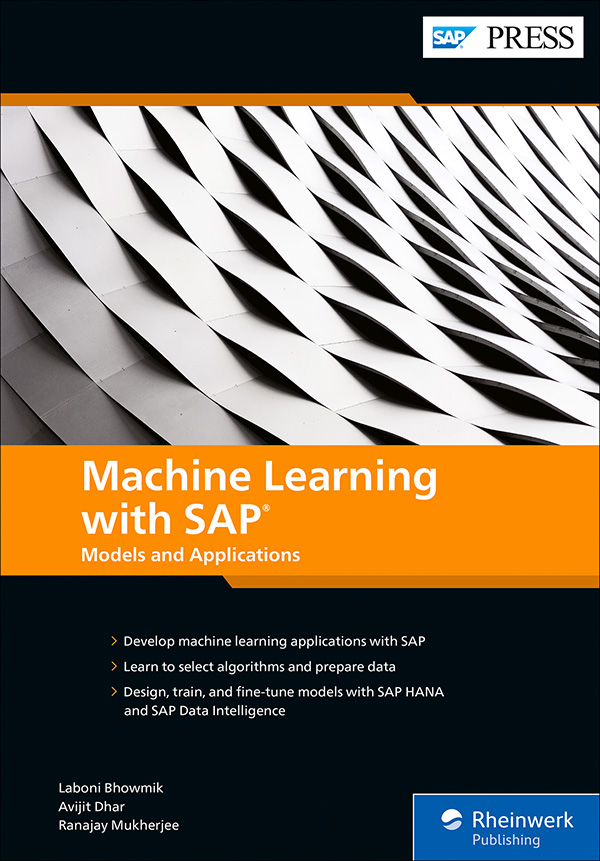Machine Learning with SAP: Models and Applications

Work smarter with machine learning! Begin with core machine learning concepts—types of learning, algorithms, data preparation, and more. Then use SAP Data Intelligence, SAP HANA, and other technologies to create your own machine learning applications. Master the SAP HANA Predictive Analysis Library (PAL) and machine learning functional and business services to train and deploy models. Finally, see machine learning in action in industries from manufacturing to banking.